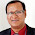### What is the difference between conjecture and hypothesis?

Similarities.
Both of them are statements based on observations, that seem to be true, but have not been proved or disproved jet.

Differences.
As explained in G.Pólya and L.Bowden book "Mathematical methods in science", difference is in level of credibility that explanation is based on. Hypothesis is testable, and explanation is based on accepted grounds. Conjecture is proposition based on inconclusive grounds, and sometimes can not be fully tested.

Another difference is that conjectures, unlike hypothesis, are used in math. Hypothesis usualy are used elsewhere. If conjecture is proven, it becomes a theorem. Still, the border between two terms remains blurry, and terms sometimes are used as synonyms. For example in math there is a conjecture called 'Riemann hypothesis', which correctly should be called ‘Riemann conjecture’.Anonymous said…
so if I get a get a geometry test and asks to name a conjecture of a statement how would I do it?Anonymous said…
Wha was that?In Popperian epistemological view, formulating a conjecture and refutation is a process of falsification. The more a conjecture is falsifiable, the more strong is the base of a theorem.In this perspective, you may compare a conjecture with a null hypothesis.